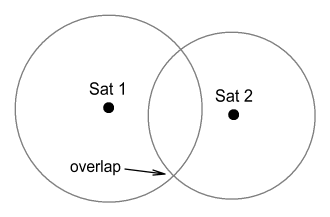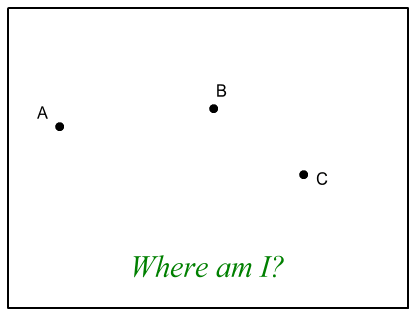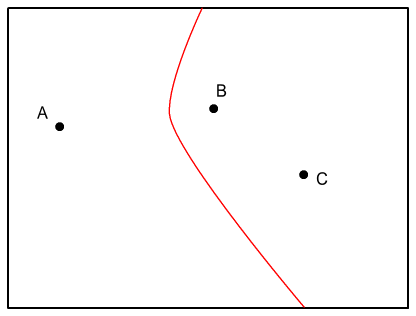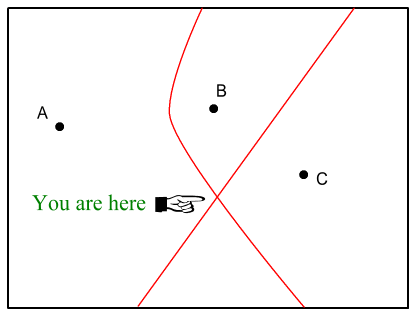Home

# GPS, Relativity, andpop-Science Mythology

Popular science frequently promotes the idea that the Global Positioning System serves not only as a means of position determination but also as a convenient test for Einstein’s Theory of Relativity.  The claim made is that if certain adjustments were not made to counter the predictions of this theory, calculated positions would quickly become very inaccurate.  Hence anyone with a functioning GPS receiver has already confirmed the theory to be correct and the pizza delivery guy would not have arrived at your home were it not for the adjustments.

The claim however is false and the science gurus who promote it should know better than to do so.  Fact is, it would not matter whether such adjustments were made or not.  Why?  The reason has to do with basic facts about how GPS receivers work.

To understand this we first need examine the claims more specifically. The following points are made in regards to Relativity and GPS operation :

1. Relativity theory predicts the clocks aboard the GPS satellites will run faster than clocks on Earth by about 38 microseconds a day.
2. The satellites’ clocks are slowed down by this amount so as to match the speed of clocks on Earth
3. If these adjustments were not made, position determination would be inaccurate by up to 11 km a day
4. This inaccuracy would grow steadily larger each day, e.g. after 10 days the inaccuracy would have grown to 110 km.

We’ll begin by assuming the first two points are absolutely correct since this essay is not about questioning the truth about relativity theory, only its application.

Odd as it may sound, the third and fourth points do not follow from the first two.

#### Determining your position

The basics of how GPS receivers work is fairly straightforward.  An array of orbiting satellites surrounds Earth.  Each satellite holds one or more highly-accurate atomic clocks.  As they orbit, a satellite transmits the time signal (or ‘timestamp’) of its on-board clock.  Since these signals travel at a finite speed – the speed of light – we can determine how far away each satellite is from the receiver and thus figure out where we are located.

Calculating the distance from a satellite requires subtracting the time signal of the satellite from the time at the receiver.  By subtracting these numbers and multiplying by the speed of light we calculate distance, i.e.

D = (tr - ts) c

Where tr is time at the receiver, ts is the (delayed) time signal from the satellite, and D is distance.  By determining the distance from at least 4 satellites we can pinpoint our location.  This calculation is generally drawn in terms of a set of overlapping spheres:In three dimensions, our position will be given by the intersection of four such spheres.

Now here’s where time dilation comes in.  If the clocks on Earth ran at a different rate to those on satellites then the above calculation would be completely out because |tr-ts| would become increasingly larger each day.  We can calculate the daily distance error δD by multiplying the daily time error δt by the speed of light:

δD = δt c ~= 38x10-6 * 3x108 = 11400 m

Or about 11 km per day.

This argument appears quite solid.  So what are we overlooking?

What we are overlooking is the phrase ‘time at the receiver’.  Problem is, GPS receivers contain no atomic clock because there’s no room to fit one in.  Plus it would be very expensive even if possible.  That ‘time at the receiver’ must instead be determined from the satellites’ clocks.  Therefore if the satellites’ clocks were running faster (or slower) than those on Earth, this ‘time at the receiver’ would likewise run faster or slower by exactly the same degree.  Hence there would be no position error due to time dilation, and certainly no cumulative position error.

Essentially, what the GPS receiver is trying to determine is not just its position but also its timestamp.  To state this mathematically, it is trying to determine variables x,y,z, and t.  That’s 4 variables which require 4 pieces of data to solve for, and the data for solving it comes from the timestamps of 4 satellites.  The receiver computes all 4 of these variables x,y,z,t but then can discard the time variable (t) as unimportant.

#### A simpler approach

Putting aside whatever method it is that GPS receivers actually use, there is a much simpler approach to calculating position that sidesteps the whole ‘time at the receiver’ question and makes it clear why the clocks’ speed relative to Earth is unimportant.  It involves comparing differences between received signals.

Let’s say you are at an unknown location on Earth (or in space) and you receive two identical signals from different satellites.  That is, the timestamps from each satellite are exactly the same when they reach you.  What would you conclude from this?  Quite obviously: that you are at a position equally distant from both satellites.  Therefore you must be somewhere on a plane that slices midway between the two satellites and runs at right-angles to a line joining them.  Using this information you have narrowed your location down to a two-dimensional plane.  And you did so without knowing your local time.  Now quite obviously also, it wouldn’t matter if those satellites’ clocks were both running faster or both running slower than yours because your equidistance makes their timestamps identical when they reach you.

That much is straightforward.  So what about a situation where there is a measured difference between time signals, as will normally be the case?  In this case it is slightly more complex but again the answer will depend only on the difference between the signals rather than any local clock’s time.

To simplify this, suppose we have a two-dimensional situation containing three satellites: A, B and C.  All satellites are at known locations with their clocks perfectly synchronised and running at identical speeds.We are somewhere in this 2D region shown and wish to determine our position.  We receive signals from A and B, and find that B is slightly ahead of A.  We subtract the time difference between these two signals and call it Δt.  What this tells us is that we are closer to B than A, and at a location where the difference between our distance to A and our distance to B is  c Δt.  Putting aside the math, this places us somewhere along a line looking like this:The line looks a bit like a parabola, although it isn’t .  Next we look at the signals from B and C and find they are identical.  As discussed above, this places us on a straight line running midway between B and C like so:Where these two lines intersect, that’s our position!

The above is a two dimensional situation.  Extending it to three dimensions will require four satellites and the intersection of three overlapping ‘parabolic’ cones.  A little more complex but the method is the same.

The important point here is that we were able to determine our location without making any reference to ‘local time’.  Doing so only required knowing of differences between satellite timestamps rather than the timestamps themselves.  So long as the satellites’ clocks all run at the same rate, these timestamp differences will be independent of the actual clock speeds.

That last point might be confusing.  After all, if I double the speed of two clocks won’t that also double the magnitude of their difference?

Short answer: no.  To easily demonstrate we’ll take two simple bench clocks, labelled A and B, and set one 10 minutes ahead of the other.  We’ll start our experiment when clock A reads 1:00 and clock B reads 1:10.  We’ll then observe them every 5 minutes and compare them to ‘real time’, which in this case will also be clock A’s time.

 real time 1:00 1:05 1:10 1:15 clock A 1:00 1:05 1:10 1:15 clock B 1:10 1:15 1:20 1:25

OK, no surprises there: B stays always 10 minutes ahead of A.

Now we redo the experiment but this time make an adjustment to both clocks so as they run at double their normal speed.  That is, for each one minute of real time, A and B move ahead 2 minutes.  We again start the experiment when A = 1:00, B = 1:10 and observe them every 5 minutes.

 real time 1:00 1:05 1:10 1:15 clock A 1:00 1:10 1:20 1:30 clock B 1:10 1:20 1:30 1:40

So the clocks move at double the rate of real time but keep the same 10 minute difference as before.

The above experiment could instead be done by having A and B set to the same time and then sending B 10 light-minutes away – about double the Earth-Mars distance.  In this case a time signal from B would take 10 minutes to reach here, meaning that its signal would read 10 minutes behind A.  Using the same reasoning as above it should be clear that doubling the speed of both clocks would not change the observed time difference (of 10 minutes) between the two.  The same goes for GPS satellites.  Whether they run faster or slower than our clocks, it will not alter their measured time differences.  And hence our GPS-calculated position will be the same.

#### Determining a Satellite’s position

Now admittedly there is an aspect in which relativity can become relevant.  And this has to do with figuring out where a satellite is.

The above exercises assume the satellites to be at fixed locations, which is not the case.  The satellites are of course in orbit, changing position all the time, and it’s up to the receivers to determine their location.  This calculation will be based on a satellite’s orbital characteristics (which are also transmitted) and its timestamp.  Essentially the timestamp allows us to determine how far around in an orbit a satellite is.  Therefore any inaccuracy in a timestamp – such as a relativistic slow-down – will cause an inaccurate position to be calculated.

Fortunately such inaccuracies will be mild because the satellites move much slower than light.  The satellites move at 3870m/s and a 38.6 microseconds-per-day inaccuracy would yield a position error of only 15cm.  Naturally this will accumulate as the days go by, but the orbital times are calculated relative to a ‘reference epoch’ which is reset weekly and the regular timestamp extends plus or minus 3.5 days from this reference time .  Hence the satellite’s calculated position could be out by up to 52cm, which is still within the normal GPS inaccuracy of 2-3 metres.  And since satellites are moving in different directions even this error should largely cancel; especially when averaged over several readings.

#### Latitudes and Altitudes

Ignoring all the above arguments for the moment, there is another important consideration that warrants attention.  And that is the fact that such relativistic effects would render the entire navigation system unworkable, at least in its present configuration.  According to the US-DOD  the satellites lose 7200 ns a day due to special relativity and gain 45900 ns a day due to general relativity.  These effects combine to yield a net speeding up of 38600 ns a day (45900-7200=38600), relative to clocks on Earth.  The clocks are then slowed by a suitable degree to negate this daily amount, and everything is fine for GPS receivers.

However since the Earth is rotating, the speed of a satellite relative to a GPS receiver will be different at different latitudes.  Therefore the amount of dilation should also be different.  That 7200 ns was calculated relative to a pole, where the Earth is not moving and only the satellite’s speed of 3870 m/s need be considered.  If the calculation was done at the equator where the surface moves at 465 m/s and GPS satellites cross at 55 degrees, the SR time dilation works out to 6300 ns.  That’s a 900 ns difference between pole and equator which presumably would yield an increasingly inaccurate position determination of 270 meters per day.

Next we have a problem with altitude because the 45900 ns figure was calculated for sea level.  This figure will decrease as we move higher and experience less gravity.  For example, at 1060 metres above sea level there will be a decrease of 10 ns per day.  This presumably would lead to an inaccuracy of 3 metres per day, accumulating up to 1 km per year.  Aeroplanes flying at 10km would be worse off; accumulating a 10 km error per year.

If it were necessary to accommodate for relativity at different latitudes and altitudes, the calculations would be very complex and would need to be done at the receiver.  But they aren’t done there: only a fixed time adjustment is made at the satellites for all points on Earth.  This fact alone demonstrates that Relativity is irrelevant to the GPS operation.

#### Conclusion

The presence of Special and General Relativity effects has no bearing on the accuracy of GPS operation.  In summary, it wouldn’t matter whether clocks aboard GPS satellites ran faster or slower than Earth’s clocks or even changed their speed each day.  Just so long as the satellites’ clocks remained synchronised with each other and the time-difference relative Earth’s clocks didn’t become too large, GPS receivers would continue to calculate their correct position.

The GPS is certainly an excellent navigational aid.  But from an operational viewpoint at least, it doesn’t serve as a test for Relativity.  Scientists should stop calling it that.

The next chapter discusses ‘secondary relativistic effects’:

GPS Mythology - part 2  (<-- click to read)

  http://en.wikipedia.org/wiki/Error_analysis_for_the_Global_Positioning_System
  The shape looks like the function y=sqrt[1+x2]
  http://www.navcen.uscg.gov/pubs/gps/sigspec/gpssps1.pdf  PDF-page 43
  http://www.aticourses.com/global_positioning_system.htm

Copyright © 2014 Bernard Burchell, all rights reserved.